# Conva

How many liters of water fit into the shape of a cylinder with a bottom diameter 20 cm and a height 45 cm?

x =  14.1372 l

### Step-by-step explanation: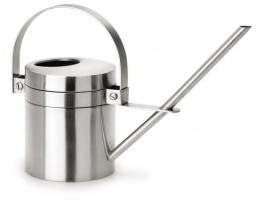Did you find an error or inaccuracy? Feel free to write us. Thank you!Tips to related online calculators
Do you know the volume and unit volume, and want to convert volume units?

## Related math problems and questions:

• Collect rain waterThe garden water tank has a cylindrical shape with a diameter of 80 cm and a height of 12 dm. How many liters of water will fit into the tank?
• The cuboidThe cuboid is full to the brim with water. The external dimensions are 95 cm, 120 cm, and 60 cm. The thickness of all walls and the bottom is 5 cm. How many liters of water fit into the cuboid?
• Children poolThe bottom of the children's pool is a regular hexagon with a = 60 cm side. The distance of opposing sides is 104 cm, the height of the pool is 45 cm. A) How many liters of water can fit into the pool? B) The pool is made of a double layer of plastic film
• Liters in cylinderDetermine the height at which level 24 liters of water in a cylindrical container having a bottom diameter 36 cm.
• Water overflow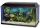A rectangular container that has a length of 30 cm, a width of 20 cm, and a height of 24 cm is filled with water to a depth of 15 cm. When an additional 6.5 liters of water are poured into the container, some water overflows. How many liters of water over
• Water cylinderZuzana poured 785 ml of water into a measuring cylinder with a base radius of 5 cm. The water in the cylinder reached a height of 2 cm from the upper edge of the cylinder. How tall is the cylinder? (π = 3.14)
• The potDiameter of the pot 38 cm. The height is 30 cm. How many liters of water can fit in the pot?
• Water levelThe glass container has a cuboid shape with dimensions of the bottom 24 cm and 12 cm. The height of the water is 26 cm. Calculate the body's volume, which is sunk into the water if the water level rise by 3 cm.
• Cylinder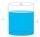The cylinder-shaped container has 80 liters of water. The water reaches 45 cm height. How many water liters will be in a container if the water level extends to a height of 72 cm? Write the result in liters, write down only as a whole or decimal number.
• Concrete boxThe concrete box with walls thick 7 cm has the following external dimensions: length 1.8 m, width 44 cm and height 46 cm. How many liters of soil can fit if I fill it to the brim?
• Snails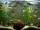How many liters of water will fit in an aquarium with bottom dimensions of 30 cm and 25 cm and a height of 60 cm, if we pour water up to a height of 58 cm? How many most snails can we keep in an aquarium, if we know that snails need 600 cm ^ 3 of water fo
• Garden pondConcrete garden pond has bottom shape of a semicircle with a diameter 1.7 m and is 79 cm deep. Daddy wants make it surface. How many liters of water is in pond if watel level is 28 cm?
• AquariumThe box-shaped aquarium is 40 cm high; the bottom has dimensions of 70 cm and 50 cm. Simon wanted to create an exciting environment for the fish, so he fixed three pillars to the bottom. They all have the shape of a cuboid with a square base. The base edg
• The potThe pot is a cylinder with a volume of V = 7l and an inner diameter of d = 20cm. Find its depth.
• Water levelHow high reaches the water in the cylindrical barell with a diameter of 12 cm if there is a liter of water? Express in cm with an accuracy of 1 decimal place.
• The inflated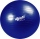The inflated gymnastic ball should have a diameter of 65 cm. How many times do we have to pump air into a fully blown ball with a bicycle inflator to inflate it if the working volume of the inflator is a cylinder with an inner diameter of 2 cm and a heigh
• Jar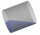From the cylinder shaped jar after tilting spilled water so that the bottom of the jar reaches the water level accurately into half of the base. Height of jar h = 7 cm and a jar diameter D is 12 cm. How to calculate how much water remains in the jar?